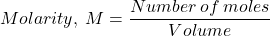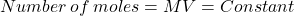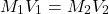Dilution is a process of adding more solvent to a certain concentrated solution to decrease its concentration. In the laboratory concentrated solutions are available often referred to as “Stock solution” which are further diluted to get the required concentration of the solution.

The concentration of a solution is expressed in terms of Molarity. We know,When more solvent is added to the solution, its volume changes and so does the molarity, but number of moles remain constant. So, we haveNumber of moles before dilution=Number of moles after dilutionLet us understand the concept of dilution more clearly while solving a numerical problem through the video.

This video covers the solution of a numerical problem: Given a stock solution of NaOH 2 mol/dm3 in 1000cm3. Work out the volume of water needed to dilute it to give 0.5 mol/dm3.

The concept of dilutions and concentrations is widely used in analytical Chemistry and forms basis of all the experiments related to Solutions.